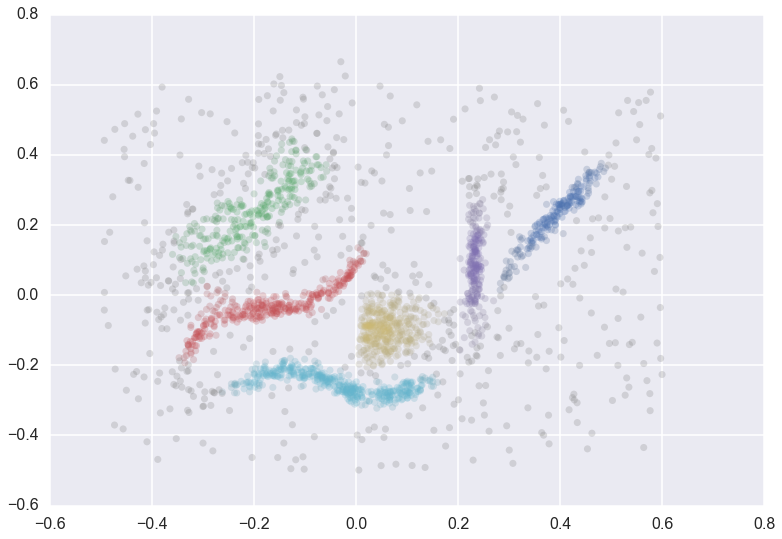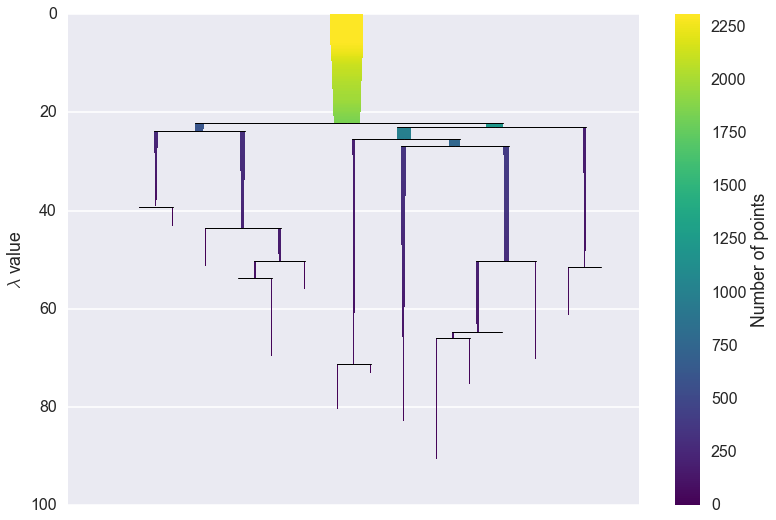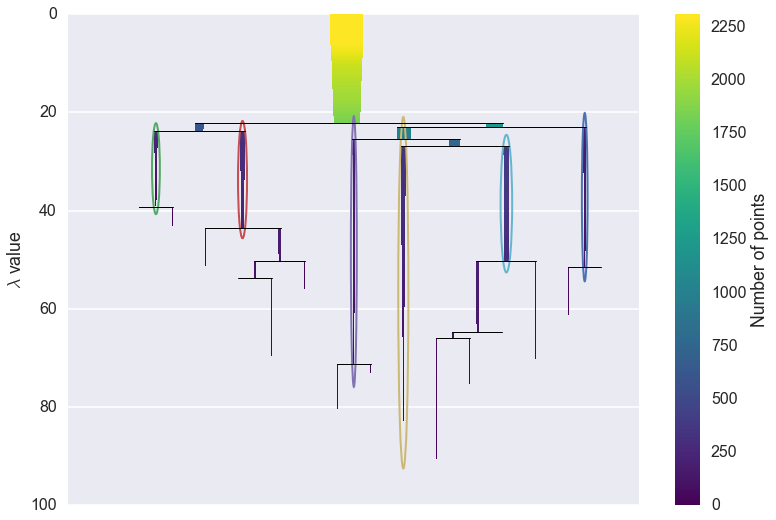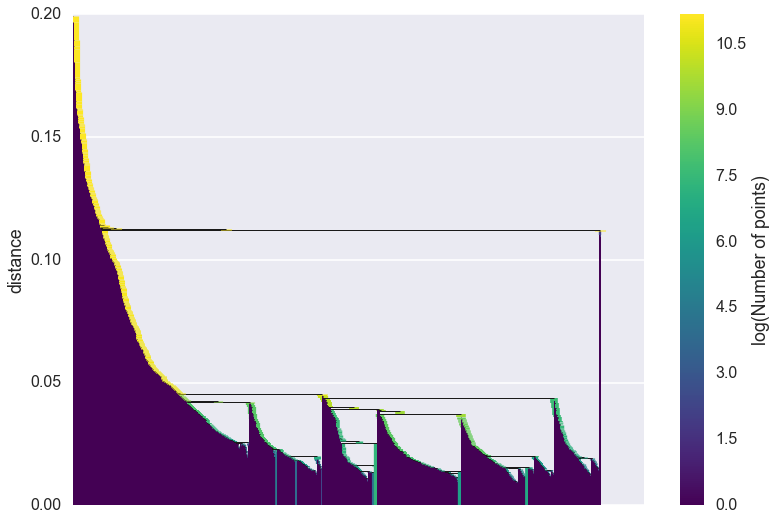Once you have the basics of clustering sorted you may want to dig a little deeper than just the cluster labels returned to you. Fortunately the hdbscan library provides you with the facilities to do this. During processing HDBSCAN* builds a hierarchy of potential clusters, from which is extracts the flat clustering returned. It can be informative to look at that hierarchy, and potentially make use of the extra information contained therein.

Suppose we have a dataset for clusteringWe can cluster the data as normal, and visualize the labels with different colors (and even the cluster membership strengths as levels of saturation)

```clusterer = hdbscan.HDBSCAN(min_cluster_size=15).fit(data)
color_palette = sns.color_palette('deep', 8)
cluster_colors = [color_palette[x] if x >= 0
else (0.5, 0.5, 0.5)
for x in clusterer.labels_]
cluster_member_colors = [sns.desaturate(x, p) for x, p in
zip(cluster_colors, clusterer.probabilities_)]
plt.scatter(*data.T, s=50, linewidth=0, c=cluster_member_colors, alpha=0.25)
```## Condensed Trees¶

The question now is what does the cluster hierarchy look like – which clusters are near each other, or could perhaps be merged, and which are far apart. We can access the basic hierarchy via the `condensed_tree_` attribute of the clusterer object.

```clusterer.condensed_tree_
```
```<hdbscan.plots.CondensedTree at 0x10ea23a20>
```

This merely gives us a CondensedTree object. If we want to visualize the hierarchy we can call the `plot` method:

```clusterer.condensed_tree_.plot()
```We can now see the hierarchy as a dendrogram, the width (and color) or each branch representing the number of points in the cluster at that level. If we wish to know which branches were selected by the HDBSCAN* algorithm we can pass `select_clusters=True`. You can even pass a selection palette to color the selections according to the cluster labelling.

```clusterer.condensed_tree_.plot(select_clusters=True,
selection_palette=sns.color_palette('deep', 8))
```From this we can see, for example, that the yellow cluster, at the center of the plot, forms early (breaking off from the pale blue and purple clusters) and persists for a long time. By comparison the green cluster, which also forming early, quickly breaks apart and then vanishes altogether (shattering into clusters all smaller than the `min_cluster_size` of 15).

You can also see that the pale blue cluster breaks apart into several subclusters that in turn persist for quite some time – so there is some interesting substructure to the pale blue cluster that is not present, for example, in the dark blue cluster.

In this was a simple visual analysis of the condensed tree can tell you a lot more about the structure of your data. This is not all we can do with condensed trees however. For larger and more complex datasets the tree itself may be very complex, and it may be desireable to run more interesting analytics over the tree itself. This can be achieved via several converter methods: `to_networkx`, `to_pandas`, and `to_numpy`.

First we’ll consider `to_networkx`

```clusterer.condensed_tree_.to_networkx()
```
```<networkx.classes.digraph.DiGraph at 0x11d8023c8>
```

As you can see we get a networkx directed graph, which we can then use all the regular networkx tools and analytics on. The graph is richer than the visual plot above may lead you to believe however:

```g = clusterer.condensed_tree_.to_networkx()
g.number_of_nodes()
```
```2338
```

The graph actually contains nodes for all the points falling out of clusters as well as the clusters themselves. Each node has an associated `size` attribute, and each edge has a `weight` of the lambda value at which that edge forms. This allows for much more interesting analyses.

Next we have the `to_pandas` method, which returns a panda dataframe where each row corresponds to an edge of the networkx graph:

```clusterer.condensed_tree_.to_pandas().head()
```
parent child lambda_val child_size
0 2309 2048 5.016526 1
1 2309 2006 5.076503 1
2 2309 2024 5.279133 1
3 2309 2050 5.347332 1
4 2309 1992 5.381930 1

Here the `parent` denotes the id of the parent cluster, the `child` the id of the child cluster (or, if the child is a single data point rather than a cluster, the index in the dataset of that point), the `lambda_val` provides the lambda value at which the edge forms, and the `child_size` provides the number of points in the child cluster. As you can see the start of the dataframe has singleton points falling out of the root cluster, with each `child_size` equal to 1.

Finally we have the `to_numpy` function, which returns a numpy record array:

```clusterer.condensed_tree_.to_numpy()
```
```array([(2309, 2048, 5.016525967983049, 1),
(2309, 2006, 5.076503128308643, 1),
(2309, 2024, 5.279133057912248, 1), ...,
(2318, 1105, 86.5507370650292, 1), (2318, 965, 86.5507370650292, 1),
(2318, 954, 86.5507370650292, 1)],
dtype=[('parent', '<i8'), ('child', '<i8'), ('lambda_val', '<f8'), ('child_size', '<i8')])
```

This is equivalent to the pandas dataframe, but is in pure numpy and hence has no pandas dependencies if you do not wish to use pandas.

We have still more data at our disposal however. As noted in the How HDBSCAN Works section, prior to providing a condensed tree the algorithm builds a complete dendrogram. We have access to this too via the `single_linkage_tree` attribute of the clusterer.

```clusterer.single_linkage_tree_
```
```<hdbscan.plots.SingleLinkageTree at 0x121d4b128>
```

Again we have an object which we can then query for relevant information. The most basic approach is the `plot` method, just like the condensed tree.

```clusterer.single_linkage_tree_.plot()
```As you can see we gain a lot from condensing the tree in terms of better presenting and summarising the data. There is a lot less to be gained from visual inspection of a plot like this (and it only gets worse for larger datasets). The plot function support most of the same fucntionality as the dendrogram plotting from `scipy.cluster.hierarchy`, so you can view various truncations of th tree if necessary. In practice, however, you are more likely to be interested in access the raw data for further analysis. Again we have `to_networkx`, `to_pandas` and `to_numpy`. This time the `to_networkx` provides a direct networkx version of what you see above. The numpy and pandas results conform to the single linkage hierarchy format of `scipy.cluster.hierarchy`, and can be passed to routines there if necessary.